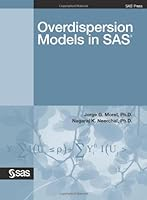# Overdispersion Models in SAS## Book Description

Overdispersion in provides a friendly methodology-based introduction to the ubiquitous phenomenon of overdispersion. A basic yet rigorous introduction to the several different overdispersion models, an effective omnibus test for model adequacy, and fully functioning commented SAS codes are given for numerous examples. The examples, many of which use the GLIMMIX, GENMOD, and NLMIXED procedures, cover a variety of fields of application, including pharmaceutical, care, and consumer products. The book is ideal as a textbook for an MS-level introductory course on estimation methods for overdispersion and generalized linear models as well as a first reading for students interested in pursuing this fertile area of research for further study. Topics covered include quasi-likelihood models; likelihood overdispersion binomial, Poisson and multinomial models; generalized overdispersion linear models (GLOM); goodness-of-fit for overdispersion binomial models; Kappa ; marginal and conditional models; generalized estimating equations (GEE); ratio estimation; small sample bias correction of GEE; generalized linear mixed models (GLMM); and generalized linear overdispersion mixed models (GLOMM).

Chapter 1 Overdispersion
Chapter 2 Generalized Linear Models
Chapter 3 Quasi-likelihood
Chapter 4 Likelihood Models for Overdispersed Binomial Responses
Chapter 5 Goodness-of-fit Tests for Overdispersed Binomial Models
Chapter 6 Likelihood Models for Overdispersed Count Responses
Chapter 7 Likelihood Models for Overdispersed Multinomial Responses
Chapter 8 A Two-stage Maximum Likelihood Estimation Procedure
Chapter 9 Generalized Estimating Equations Techniques for Marginal Models
Chapter 10 Generalized Linear Overdispersion Mixed Models